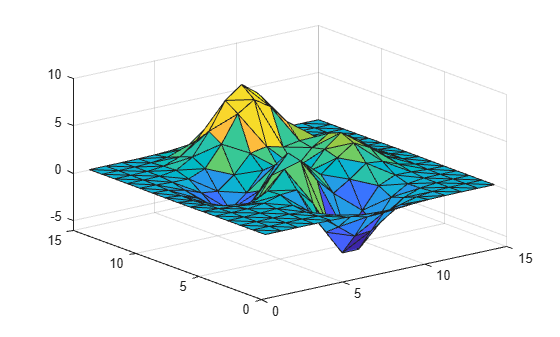Documentation

# trisurf

Triangular surface plot

## Syntax

```trisurf(Tri,X,Y,Z,C) trisurf(Tri,X,Y,Z) trisurf(TR) trisurf(...'PropertyName',PropertyValue...) h = trisurf(...) ```

## Description

`trisurf(Tri,X,Y,Z,C) ` displays triangles defined in the m-by-3 face matrix `Tri` as a surface. Each row of `Tri` defines a single triangular face by indexing into the vectors or matrices that contain the `X`, `Y`, and `Z` vertices. The color is defined by the vector `C`.

`trisurf(Tri,X,Y,Z)` uses `C=Z` so color is proportional to surface height.

`trisurf(TR)` displays the triangles in a triangulation representation. It uses `C = TR.Points(:,3)` to make sure the surface color is proportional to height.

`trisurf(...'PropertyName',PropertyValue...) ` specifies additional patch property names and values for the patch graphics object created by the function.

`h = trisurf(...)` returns a patch handle.

## Examples

collapse all

Create vertex vectors and a face matrix, then create a triangular surface plot.

```[x,y] = meshgrid(1:15,1:15); tri = delaunay(x,y); z = peaks(15); trisurf(tri,x,y,z)```If the surface is in the form of a triangulation representation, you can pass it to `trisurf` alone:

```tr = triangulation(tri,x(:),y(:),z(:)); trisurf(tr)```Download ebook